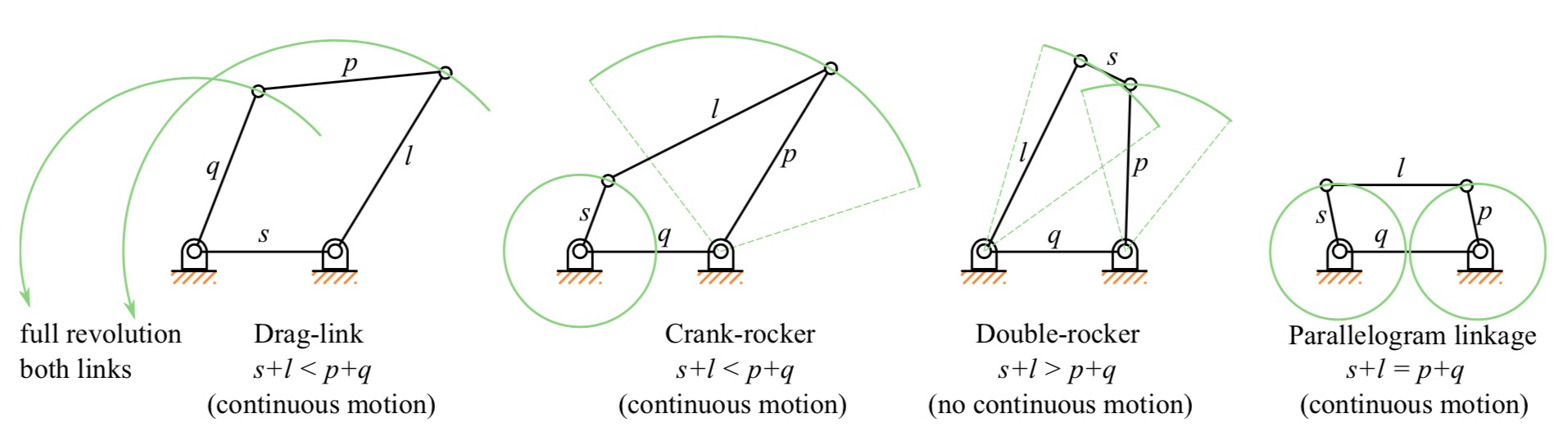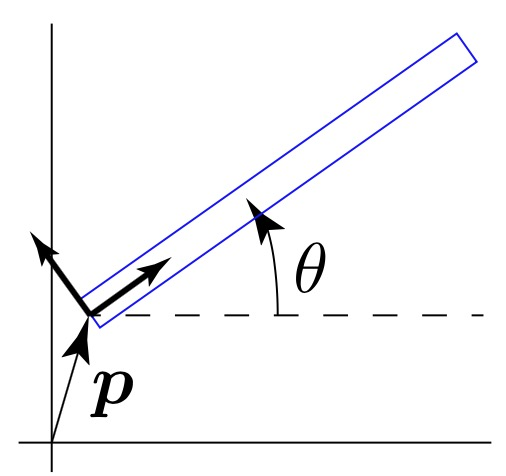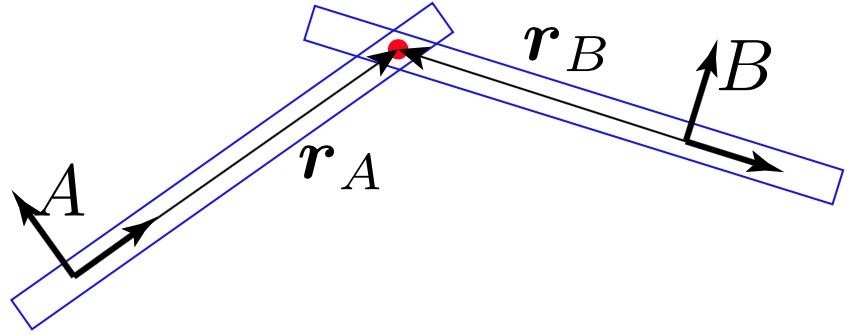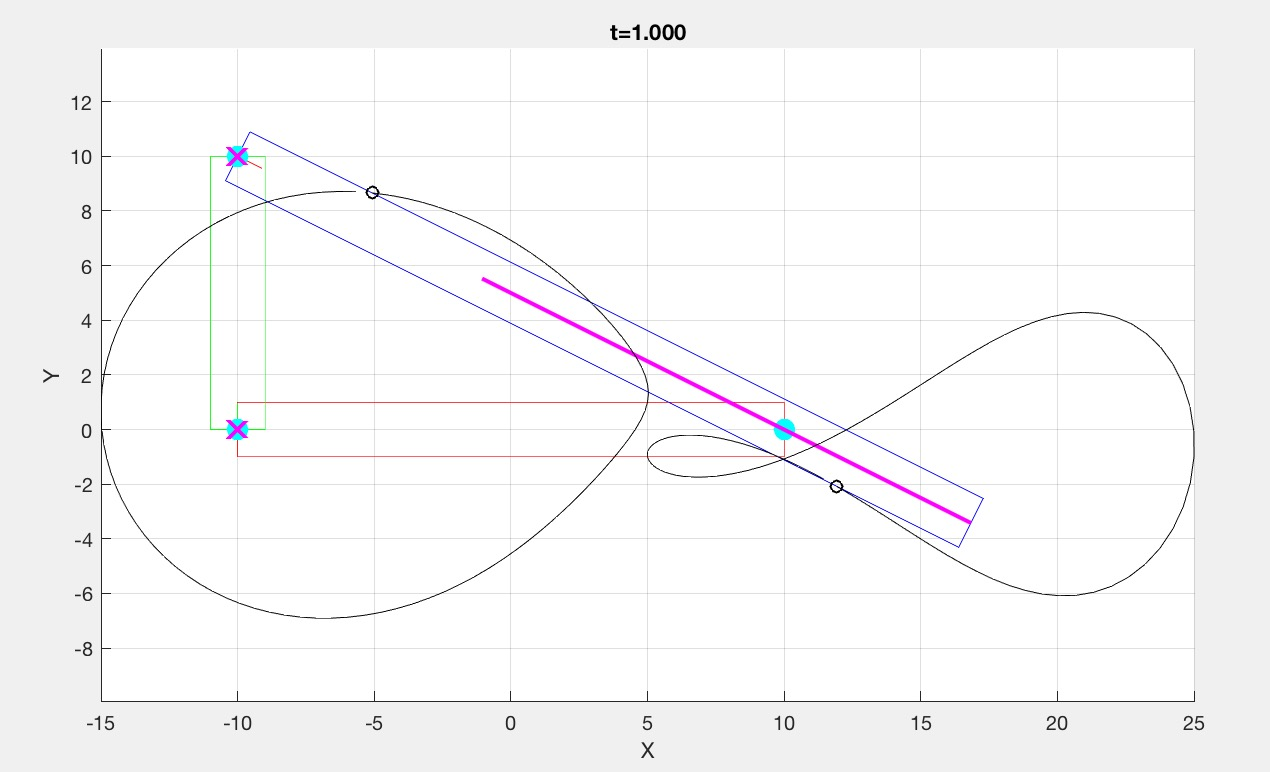# Assignment 5 - Computational Linkage Design

Due Sunday 10/29 at 11:59 pm. You must work individually.

## Overview

A linkage is an assembly of bodies connected to manage forces and movement. In this assignment you will design some interesting linkages, which can be physically manufactured. We will be only looking at kinematics and ignore forces, and furthermore, we will limit ourselves to 2D planar structures with revolute joints. Provided with this assignment is a fully functional Matlab code for simulating linkages.

Here are some sample linkages.Image source: Wikipedia. s = smallest link length; l = longest link length; p, q = other two lengths.

In this assignment you will be responsible for:

1. Adding tracer particles for display.
2. Modifying the code to allow oscillatory driver input (instead of rotational).
3. Designing several other linkages.
4. Adding support for slider mechanisms.

## Running the Code

Download the Matlab base code. The provided code is standalone and does not require any external libraries. Copy or move linkages.m to WORKINGDIR. Then open Matlab, go to the command window, and type:

>> cd <WORKINGDIR>
>> linkages(0)

Matlab will then open a figure window that shows the simulation of the sample linkage. The pin constraint between the right and top links is randomized, so if you run the code multiple times, you will get a slightly different mechanism each time. The argument 0 specifies the scene to run, which in this case is a crank-rocker mechanism. Each new linkage mechanism that you create should have a new scene number.

Note: You will also need the Optimization Toolbox, which should be available from TAMU through whatever link you used to obtain Matlab.

## How to Create ScenesA link defined by its orientation, \theta (a scalar), and position, p (2D vector).

To create a scene, we need a list of links and a list of constraints between the links. These are called links and pins in the code. Scene creation is done inside the switch statement at the top of the code. The sample four-bar linkage scene is defined in case 0.

A link is modeled as a rigid body, and in 2D, it has three degrees of freedom: \theta \in R and p \in R^2 (see figure above). \theta specifies the orientation of the link with respect to the positive x-axis, and p specifies the position of the center of rotation. Given a 2D point, r, in the link's local coordinates, the world coordinates of that point can be computed as

x = R(\theta) \, r + p,

where R is the 2x2 rotation matrix given by

R = \begin{pmatrix} \cos \theta & -\sin \theta\\ \sin \theta & \cos \theta \end{pmatrix}.

For example, the following code creates a horizontal link at the origin.

links(1).angle = 0;
links(1).pos = [0,0]';

The kinematics of a link is fully expressed using \theta and p. In order to draw it on screen, however, we need to give it a mesh. In this assignment, we simply assign to each link a list of vertices expressed in the link's local coordinates and transform these vertices into world coordinates whenever we move the link. Note that the mesh is for display only--the simulation would work just fine even if we do not have a mesh. To specify the vertices, we write to the verts field of the link object. For example,

links(1).verts = [
-1.0  21.0  21.0 -1.0
-1.0  -1.0   1.0  1.0
];

would define four vertices

In other words, these vectors are stored column wise in the links(1).verts matrix. Note that these four vertices need not be centered with respect to the origin of the link's frame. Since the mesh is not used for the simulation but only for the display, we can place the mesh anywhere with respect to the frame (or conversely the frame with respect to the mesh). Also note that we don't need to use rectangular shapes, as illustrated by the top link. You can add more vertices to create any shape you like.

Additionally, we specify that:

1. One of the links is grounded, meaning that it cannot move. This link is shown in red.
2. Another link is the driver, meaning that its orientation and position are specified procedurally. This link is shown in green.

For example,

grounded = 1;
driver = 2;

specifies that links(1) is grounded, and links(2) is the driver. You can set the motion of the driver to be anything. However, for this assignment, only rotational (or oscillatory) motion is needed. The easiest way to achieve this is to set the location of the pin constraint (described below) between the driver link and the grounded link to be at the origin of the driver link, and then specifying just the rotation of the driver link, keeping the position of the driver link fixed.

### Pin ConstraintsA pin between two links. The locations r_A and r_B (2D vectors)
define where the pin location is with respect to the links.

A "pin" constrains two links with a revolute joint. It stores the indices of the two links to constrain as well as where on the two links the pin constraint is located. See r_A and r_B in the figure above. The constraint should then make sure that, if r_A and r_B are transformed to world space, their locations match.

The following code creates a pin between links(1) and links(2) as shown in the figure above.

pins(1).linkA = 1;
pins(1).pointA = [4,0]';
pins(1).pointB = [-4,0]';

In this case,

r_A = \begin{pmatrix}4\\0\end{pmatrix}, \quad r_B = \begin{pmatrix}-4\\0\end{pmatrix}.

HINT: When creating scenes, the links do not need to be precisely positioned, since they will snap to configurations that satisfy all the constraints, as long as those constraints are satisfiable.

### Particles

To accentuate the interesting motions of linkages, we can add tracer particles to the links (like the green lines in the 1st figure). The following code creates a particle.

particles(1).link = 4;
particles(1).point = [0.5,0.1]';

The link field specifies the parent link, and the point field specifies where on this parent link the particle is located (expressed in the parent link's coordinates).

## How the Simulation Works

NOTE: This section is optional, but may be needed for designing slider joints (extra credit for 489, required for 689).

After the scene creation process finishes, we have a list of links, pins, and particles. The simulator then takes this information and does the following:

while simulating
1. Procedurally set the driver angle
2. Solve for linkage orientations and positions
3. Update particle positions
4. Draw scene
end

In Step 1, we manually specify the target angle of the driver link. As long as the mechanism has a single degree of freedom, the orientations and the positions of the rest of links can be solved for in Step 2 using nonlinear least squares. In Step 3, we compute the world positions of the particles given the newly updated link configurations. Finally, in Step 4, we draw the scene on the screen.

The meat of the simulator is in Step 2. The optimization variables in the nonlinear least squares problem are the orientations, \theta, and positions, p, of all the links, including grounded and driver links. We will use q_i = (\theta_i,p_i)^T to denote the configuration of the ith link. We are looking for q_1,\cdots,q_n so that all of the constraints are satisfied. There are two types of constraints implemented so far: "prescribed" and "pin". In both cases, we’ll express the constraint in the form c(q) = 0. This is a vector function--c is a concatenation of all the constraints added to the system.

#### Prescribed

Grounded and driver links have their configurations manually specified. We can express this as a constraint on the orientation \theta and the position p of the link.

c_\theta(q) = \theta - \theta_{{target}}, \quad c_p(q) = p - p_{{target}}.

Here, c_\theta(q) is a scalar, and c_p(q) is a 2D vector. (Thus, with these two constraints, c(q) is a 3x1 vector.) For grounded links, the target orientation and position are fixed throughout the simulation (i.e., \theta_{{target}} is fixed), whereas for driver links, they are specified procedurally (i.e., \theta_{{target}} changes over time).

#### Pin

A pin constrains two links so that they share a common point. Let the two links be denoted by A and B. We can express the constraint as

c_{{pin}}(q) = x_A - x_B,

where x_A and x_B are both in world coordinates, which must be computed from the local coordinates, r_A and r_B. In other words, we are looking for orientations and positions of the two links such that this constraint function evaluates to zero. The orientations and positions of the two links, \theta and p, are the variables of this constraint function, whereas the locations of the pin joints expressed in local coordinates, r_A and r_B, are the parameters of this constraint function.

### Solving

Let \vec{c}(\vec{q}) be the concatenation of all the constraints. We are looking for the orientations and positions of all the joints (\theta_i and p_i) such that the constraint violations are minimized:

\underset{\vec{q}}{\text{minimize}} \quad \frac{1}{2} \vec{c}^T \vec{c}.

We can solve this easily in Matlab using the function lsqnonlin. What we need to provide is a function that evaluates the vector-valued function \vec{c}. This is implemented in the function objFun(...) in the code. In this function, each scalar element of \vec{c} must be evaluated using the current angles and positions of the links, which is \vec{q} = (q_1,\cdots,q_n)^T = (\theta_1, p_1, \cdots, \theta_n, p_n)^T.

The optimization process can be sped up by providing the derivatives of \vec{c}, called the Jacobian, J = \frac{\partial \vec{c}}{\partial \vec{q}}. The "ground/driver" constraint and the "pin" constraint have the Jacobians implemented. You can turn on/off these Jacobians by changing the arguments to optimoptions().

• The first task is to write code to compute the world positions of the tracer particles. In the updateParticles(...) function, use the particle's local position and the parent link's current angle and position to compute the world position of the particle, x.

• Next, modify the driver so that it can also generate oscillatory motion in addition to rotational motion. (There should be a way to switch between the two.) Currently, the piece of code that produces rotational motion is

...
dt = 0.01;
angVel = 2*pi;
while t < T
...
end
...

This integrates the target angle with a constant angular velocity (2\pi radians per second), so that the driver rotates at a constant rate. Your task here is to modify this code so that you can specify a range that the driver oscillates between. For example, if the range is [−\pi/3,\pi], then the driver should go back and forth between −pi/3 and \pi at some set frequency. Hint: Use a sine function that oscillates between two values.

• Design a family of four-bar linkages. The sample code (linkages(0)) produces a crank-rocker mechanism, since the shortest link, s, is the crank (driver). Copy and paste this sample code and create a drag-link mechanism and a double-rocker mechanism. Place all the code in the appropriate case block. For the double-rocker, the driver motion must be oscillatory, not rotational.

• Choose one of the following:

• Design a Chebyshev's Lambda Mechanism. This is a crank-rocker mechanism that produces a nearly straight line. Make sure you add a tracer particle to show the trajectory of the tip.

• Design a Peaucellier-Lipkin Linkage. "This was the first planar linkage capable of transforming rotary motion into perfect straight-line motion, and vice versa." Again, make sure you add a tracer particle to show the trajectory of the tip.

• Design a Klann Linkage. See also this animation. You only need to design one limb consisting of 6 links. For this mechanism, the geometry of some of the links will no longer be rectangles. You will need to modify the contents of the verts field of link to make this change. Note that the drawScene(...) function draws the link geometry by connecting the vertices in the order they are defined.

• For 489 students: Design an additional linkage. It must be of moderate complexity, though an interesting but simple linkages are acceptable. Please ask if you are not sure. Make sure to cite any references. This additional linkage cannot be the one you did not choose for Task 4.

• For 689 students: Implement a sliding joint and create a mechanism using it. (As an illustration, see the scissor mechanism). You will have to modify, among other things, objFun(...). This function is called by lsqnonlin to evaluate the constraints. To make this modification a little easier, you should ask lsqnonlin to use finite differencing instead of using analytical gradients. (This is already done for you in case 10.) The code will be slower with finite differencing, but providing analytical gradients can be tricky business. Here are some hints on implementing this constraint:

• You may treat this slider as an infinite slider.
• Unlike the pin constraint, which takes two rows (a constraint for the x-coordinate and another constraint for the y-coordinate), the slider constraint is a scalar constraint.
• You should store not just a single point for body B but a pair of points. In other words, you'll need to add fields sliders(end).pointB1 and sliders(end).pointB2.
• The constraint should be based on the triangle area between the points A1, B1, and B2. This area should be zero. Compute the triangle area and put it into the appropriate row of \vec{c}.
• You will also need to modify the drawScene(...) function to draw the sliders.A sample scene with a slider constraint. Your scene should be more complex.

## Evaluation

For a grade of B, you must finish:

• The particle tracer
• Double-rocker
• Chebyshev or Peaucellier-Lipkin

For a grade of A, you must also finish:

• For 489 students
• Klann
• 10% extra with the slider constraint
• For 689 students
• Klann
• An additional linkage using the slider constraint

## What to hand in

Please follow these instructions carefully. If you fail to follow these instructions, you will be docked some points.

Please provide a report with your submission (PDF). The report should include the following:

• Images of all mechanisms that you designed.
• Were there any references (books, papers, websites, etc.) that you found particularly helpful for completing your assignment? Please provide a list.
• Are there any known problems with your code? If so, please provide a list and, if possible, describe what you think the cause is and how you might fix them if you had more time or motivation. This is very important, as we are much more likely to assign partial credit if you help us understand what is going on.
• Did you implement the slider? Include an image of the mechanism. If there was a substantial amount of work involved, describe what and how you did it.
• Do you have any comments about this assignment that you would like to share?

Create a zip file named NETID.zip (your email ID; e.g., sueda.zip) so that when you extract it, it creates a folder called NETID/ (e.g., sueda/) that has (ONLY) the following in it:

• Your PDF report (any filename is OK)
• Your Matlab file (linkages.m)

Generated on Wed Oct 25 09:52:42 CDT 2017